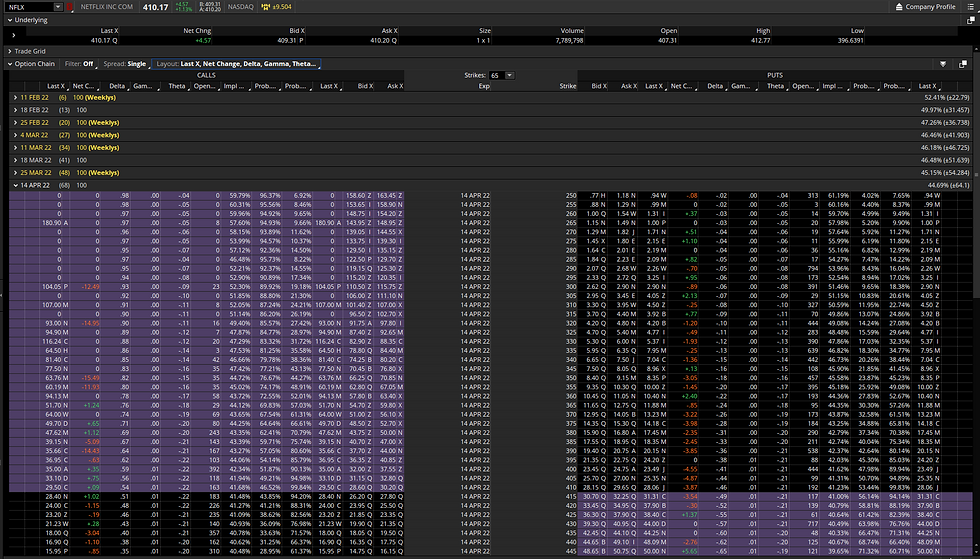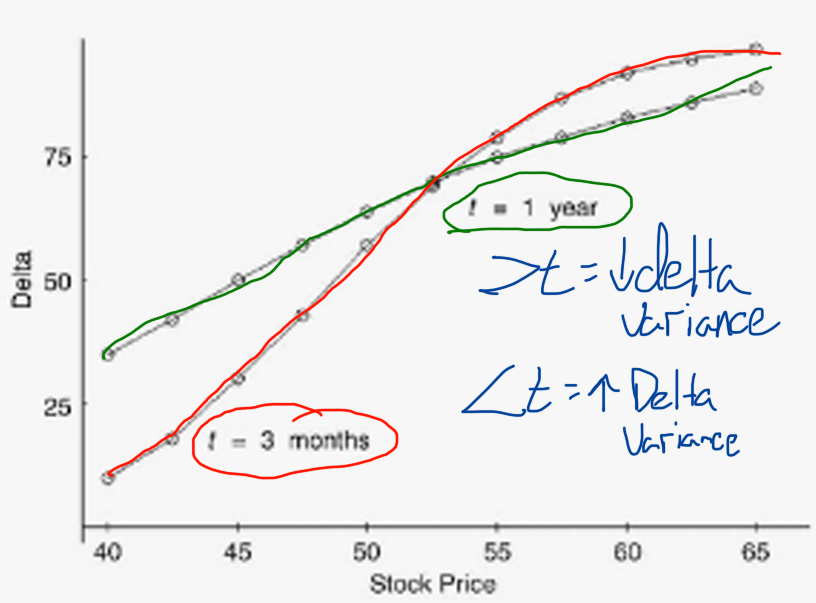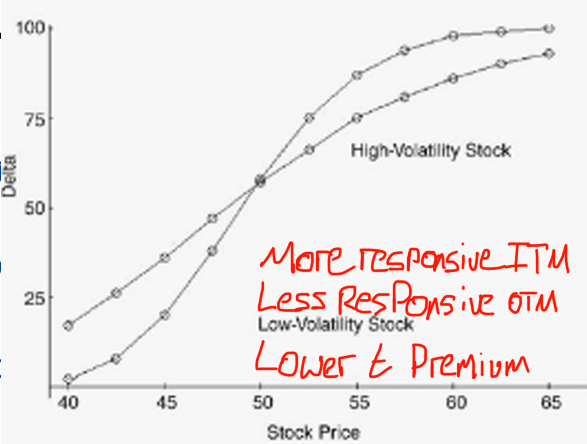top of page
Search

# Delta Explained - Option Greeks Deep Dive Pt1

Delta is one of the more diverse Greeks with a plethora of uses associated with it. In a textbook sense, delta measures the change in premium of an option for every 1 point move in the underlying. This assumes time, volatility, and interest rates all remain the same- which we know they don't.

Delta ranges from -1 (puts are negative when bought) to +1 (calls are positive when bought). We can use it for:

• It's textbook definition

• As a rough probability of an option expiring ITM

• As a rough probability of an option being touched or breached prior to expiration by multiplying delta by 2

• *Note I say rough probability - it's not an exact calculation but it's generally close enough. For example, note the disparity in the Delta and Probability of ITM calculation for NFLX below. Take the -.31 delta 380P, this has a 37.34% of being ITM at expiration, as compared to the 31% we would infer from delta (6.34% variance). You'll tend find a larger disparity between the two the further you go out in time. Looking at the 18Feb expiration, the -0.32 delta 395P has a 35.58% probability of being ITM compared to the 32% delta inferred PITM (a 3.58% variance).• We can also use delta to understand the TOTAL number of shares of the underlying we have the potential to control. A 1 lot can control up to 100 shares. A 5 lot can control up to 500 shares, etc.

• As we know, delta moves around. We can use delta to understand how many shares of the underlying our position is CURRENTLY behaving like. If we have a 0.30 delta call, we're controlling the equivalent of long 30 shares in the underlying.

• Based on the previous two, we can use delta to calculate equivalent positions for pairs trading, hedging, portfolio risk assessment, etc.

• We can also use a tool called, Beta Weighting, to translate all our current positions to a common underlying - like the SPY

Now that we know what we can use it for, let's review what impacts delta. As noted above, delta measures changes in premium. It's important to note that there are significant impacts to delta by DTE, volatility, and interest rates (for LEAPS and longer dates). I'll focus on time and volatility, the impact of rates is generally lower and we'll cover that in a future session on Rho.

• Time. Notice how shorter DTE options see far more variance in their delta (this is gamma risk). Also note how the simple passage of time erodes delta as OTM options assume towards 0 and ITM move towards 100.• Volatility. Note how lower volatility underlyings are less responsive when further OTM and accelerate when ITM. This is due to the valuation in time premium in the options, they will have lower extrinsic value due to their decreased volatility. Higher volatility underlyings will see more aggressive OTM changes in premium due to higher time premium valuations and will slow when ITM due to a decrease in time premium (due to rising extrinsic values).